Processing ......FreeComputerBooks.com Links to Free Computer, Mathematics, Technical Books all over the World

Computational And Algorithmic Linear Algebra And N-dimensional Geometry

• Title Computational And Algorithmic Linear Algebra And N-dimensional Geometry
• Author(s) Katta Gopalakrishna Murty
• Publisher: World Scientific (July 31, 2014); eBook (Internet Edition with Errata)
• Permission: Link to the Internet Edition of the book in author's homepage.
• Hardcover/Paperback 478 pages
• eBook PDF Files
• Language: English
• ISBN-10/ASIN: 9814366633/B00OAV2WCY
• ISBN-13: 978-9814366632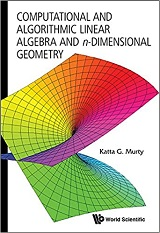Book Description

This undergraduate textbook on Linear Algebra and n-Dimensional Geometry, in a self-teaching style, is invaluable for sophomore level undergraduates in mathematics, engineering, business, and the sciences.

These are classical subjects on which there are many mathematics books in theorem-proof style, but this unique volume has its focus on developing the mathematical modeling as well as computational and algorithmic skills in students at this level.

The explanations in this book are detailed, lucid, and supported with numerous well-constructed examples to capture the interest and encourage the student to master the material.

• Katta Gopalakrishna Murty is a Professor, Industrial and Operations engineering, The University of Michigan, Ann Arbor.
Reviews, Ratings, and Recommendations: Related Book Categories: Read and Download Links:Similar Books:
•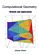Computational Geometry: Methods and Applications (Jianer Chen)

An introduction to some of the fundamental principles of computational geometry, concentrates on four major directions in computational geometry: the construction of convex hulls, proximity problems, searching problems and intersection problems.

•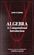Algebra: A Computational Introduction (John Scherk)

This book is a unique approach and presentation, the author demonstrates how software can be used as a problem-solving tool for algebra. It includes many computations, both as examples and as exercises.

•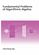Fundamental Problems of Algorithmic Algebra (Chee-Keng Yap)

This book provides a systematic and focused treatment of a collection of core problemsthe computational equivalents of the classical Fundamental Problem of Algebra and its derivatives.

•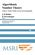Algorithmic Number Theory: Lattices, Curves, and Cryptography

This text provides a comprehensive introduction to algorithmic number theory for beginning graduate students, written by the leading experts in the field.

•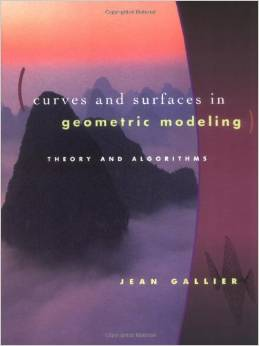Curves and Surfaces in Geometric Modeling: Theory and Algorithms

This book offers both a theoretically unifying understanding of polynomial curves and surfaces and an effective approach to implementation. It is also an exellent introduction to geometry concepts used in computer graphics, vision, robotics, geometric modeling.

•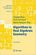Algorithms in Real Algebraic Geometry (Saugata Basu, et al)

The monograph gives a self-contained detailed exposition of the algorithmic real algebraic geometry. It will be useful both for beginners and for advanced readers, who work in real algebraic geometry or apply its methods in other fields.

•Algorithmic Algebra (Bhubaneswar Mishra)

Anyone need to use algorithms in polynomials should have a copy of this! This is definitely one of the best! It covers almost everything anyone need in computation with systems of polynomial equations: Grobner Basis, Resultants, Characteristic Set, etc.

•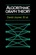Algorithmic Graph Theory (David Joyner, et al)

This is an introductory book on algorithmic graph theory. Theory and algorithms are illustrated using the Sage open source mathematics software. It's especially suitable for computer scientists and mathematicians interested in computational complexity.

•Applied and Computational Linear Algebra: A First Course

This book is intended as a text for a graduate course that focuses on applications of linear algebra and on the algorithms used to solve the problems that arise in those applications.

Book Categories
 :All CategoriesRecent BooksMiscellaneous BooksComputer LanguagesComputer ScienceData Science/DatabasesElectrical EngineeringJava and Java EE (J2EE)Linux and UnixMathematicsMicrosoft and .NETMobile ComputingNetworking and CommunicationsSoftware EngineeringSpecial TopicsWeb Programming
Other Categories